Newton’s laws of motionPage 7

WATCH ALL SLIDES

Slide 52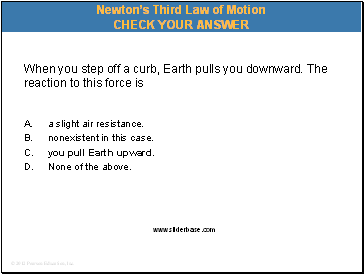When you step off a curb, Earth pulls you downward. The reaction to this force is

A. a slight air resistance.

nonexistent in this case.

you pull Earth upward.

None of the above.

Newton’s Third Law of Motion

Slide 53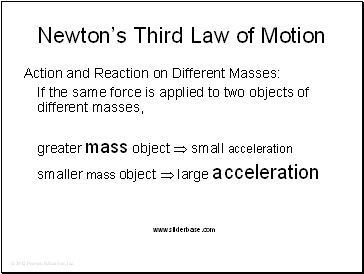Newton’s Third Law of Motion

Action and Reaction on Different Masses:

If the same force is applied to two objects of different masses,

greater mass object  small acceleration

smaller mass object  large acceleration

Slide 54When a cannon is fired, the accelerations of the cannon and cannonball are different, because the

A. forces don’t occur at the same time.

forces, although theoretically are the same, in practice aren’t the same.

masses are different.

ratios of force to mass are the same.

Newton’s Third Law of Motion

Slide 55When a cannon is fired, the accelerations of the cannon and cannonball are different, because the

A. forces don’t occur at the same time.

forces, although theoretically are the same, in practice aren’t the same.

masses are different.

ratios of force to mass are the same.

Newton’s Third Law of Motion

Slide 56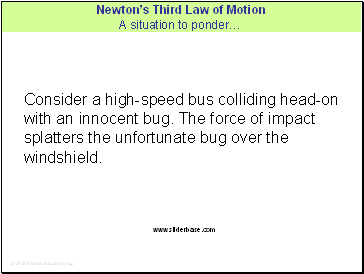Consider a high-speed bus colliding head-on with an innocent bug. The force of impact splatters the unfortunate bug over the windshield.

Newton’s Third Law of Motion

A situation to ponder…

Slide 57Which is greater, the force on the bug or the force on the bus?

A. Bug.

Bus.

Both are the same.

Cannot say.

A situation to ponder…

Slide 58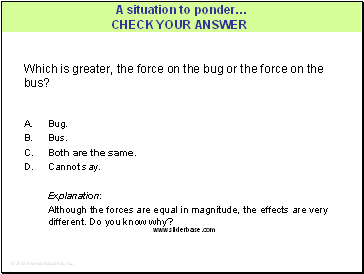Which is greater, the force on the bug or the force on the bus?

A. Bug.

Bus.

Both are the same.

Cannot say.

Explanation:

Although the forces are equal in magnitude, the effects are very different. Do you know why?

A situation to ponder…

Slide 59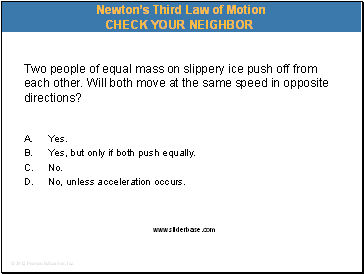Two people of equal mass on slippery ice push off from each other. Will both move at the same speed in opposite directions?

A. Yes.

Yes, but only if both push equally.

No.

No, unless acceleration occurs.

Newton’s Third Law of Motion